## Electronic Structure of Atoms (Electron Configurations)

### Learning Objectives

By the end of this section, you will be able to:

• Derive the predicted ground-state electron configurations of atoms
• Identify and explain exceptions to predicted electron configurations for atoms and ions
• Relate electron configurations to element classifications in the periodic table

Having introduced the basics of atomic structure and quantum mechanics, we can use our understanding of quantum numbers to determine how atomic orbitals relate to one another. This allows us to determine which orbitals are occupied by electrons in each atom. The specific arrangement of electrons in orbitals of an atom determines many of the chemical properties of that atom.

## Orbital Energies and Atomic Structure

The energy of atomic orbitals increases as the principal quantum number, n, increases. In any atom with two or more electrons, the repulsion between the electrons makes energies of subshells with different values of l differ so that the energy of the orbitals increases within a shell in the order s < p < d < f. Figure 1 depicts how these two trends in increasing energy relate. The 1s orbital at the bottom of the diagram is the orbital with electrons of lowest energy. The energy increases as we move up to the 2s and then 2p, 3s, and 3p orbitals, showing that the increasing n value has more influence on energy than the increasing l value for small atoms. However, this pattern does not hold for larger atoms. The 3d orbital is higher in energy than the 4s orbital. Such overlaps continue to occur frequently as we move up the chart.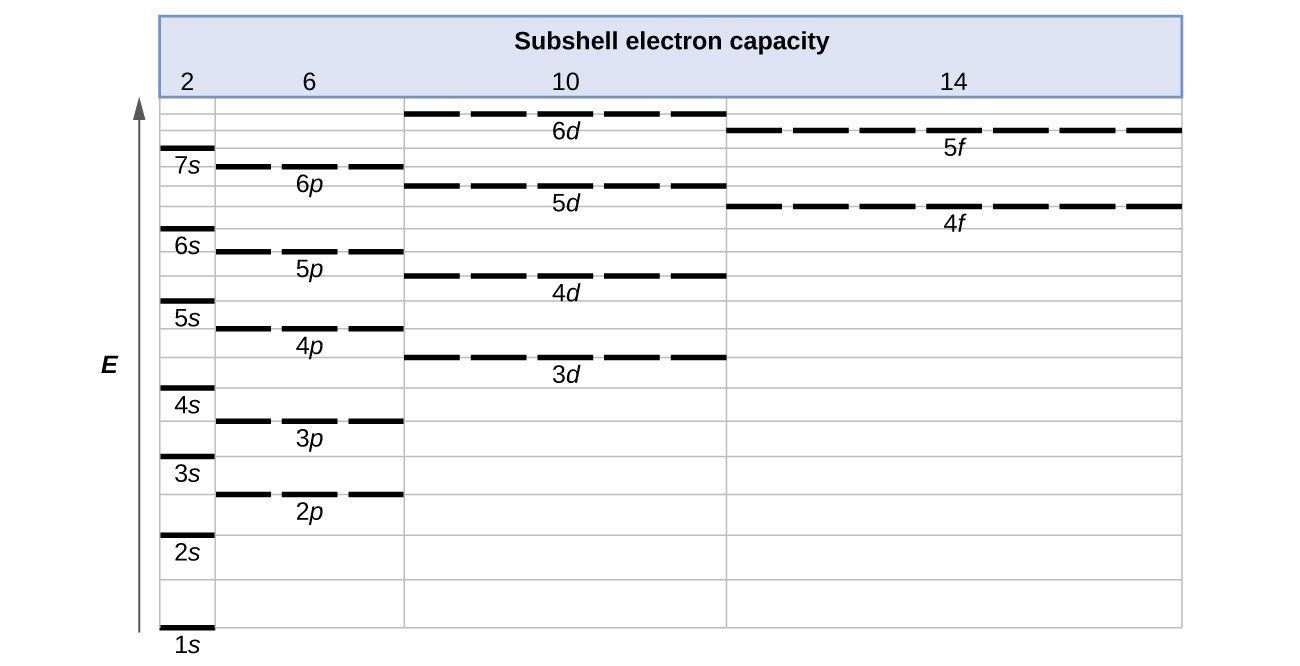Figure 1. Generalized energy-level diagram for atomic orbitals in an atom with two or more electrons (not to scale).

Electrons in successive atoms on the periodic table tend to fill low-energy orbitals first. Thus, many students find it confusing that, for example, the 5p orbitals fill immediately after the 4d, and immediately before the 6s. The filling order is based on observed experimental results, and has been confirmed by theoretical calculations. As the principal quantum number, n, increases, the size of the orbital increases and the electrons spend more time farther from the nucleus. Thus, the attraction to the nucleus is weaker and the energy associated with the orbital is higher (less stabilized). But this is not the only effect we have to take into account. Within each shell, as the value of l increases, the electrons are less penetrating (meaning there is less electron density found close to the nucleus), in the order s > p > d > f. Electrons that are closer to the nucleus slightly repel electrons that are farther out, offsetting the more dominant electron–nucleus attractions slightly (recall that all electrons have −1 charges, but nuclei have +Z charges). This phenomenon is called shielding and will be discussed in more detail in the next section. Electrons in orbitals that experience more shielding are less stabilized and thus higher in energy. For small orbitals (1s through 3p), the increase in energy due to n is more significant than the increase due to l; however, for larger orbitals the two trends are comparable and cannot be simply predicted. We will discuss methods for remembering the observed order.

The arrangement of electrons in the orbitals of an atom is called the electron configuration of the atom. We describe an electron configuration with a symbol that contains three pieces of information (Figure 2):

1. The number of the principal quantum shell, n,
2. The letter that designates the orbital type (the subshell, l), and
3. A superscript number that designates the number of electrons in that particular subshell.

For example, the notation 2p4 (read “two–p–four”) indicates four electrons in a p subshell (l = 1) with a principal quantum number (n) of 2. The notation 3d8 (read “three–d–eight”) indicates eight electrons in the d subshell (i.e., l = 2) of the principal shell for which n = 3.Figure 2. The diagram of an electron configuration specifies the subshell (n and l value, with letter symbol) and superscript number of electrons.

## The Aufbau Principle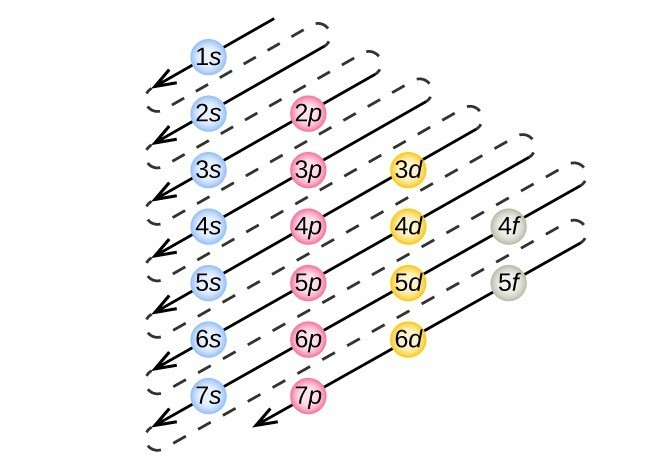Figure 3. The arrow leads through each subshell in the appropriate filling order for electron configurations. This chart is straightforward to construct. Simply make a column for all the s orbitals with each n shell on a separate row. Repeat for p, d, and f. Be sure to only include orbitals allowed by the quantum numbers (no 1p or 2d, and so forth). Finally, draw diagonal lines from top to bottom as shown.

To determine the electron configuration for any particular atom, we can “build” the structures in the order of atomic numbers. Beginning with hydrogen, and continuing across the periods of the periodic table, we add one proton at a time to the nucleus and one electron to the proper subshell until we have described the electron configurations of all the elements.

This procedure is called the Aufbau principle, from the German word Aufbau (“to build up”). Each added electron occupies the subshell of lowest energy available (in the order shown in Figure 1), subject to the limitations imposed by the allowed quantum numbers according to the Pauli exclusion principle. Electrons enter higher-energy subshells only after lower-energy subshells have been filled to capacity. Figure 3 illustrates the traditional way to remember the filling order for atomic orbitals. Since the arrangement of the periodic table is based on the electron configurations, Figure 4 provides an alternative method for determining the electron configuration. The filling order simply begins at hydrogen and includes each subshell as you proceed in increasing Z order. For example, after filling the 3p block up to Ar, we see the orbital will be 4s (K, Ca), followed by the 3d orbitals.Figure 4. This periodic table shows the electron configuration for each subshell. By “building up” from hydrogen, this table can be used to determine the electron configuration for any atom on the periodic table.

We will now construct the ground-state electron configuration and orbital diagram for a selection of atoms in the first and second periods of the periodic table. Orbital diagrams are pictorial representations of the electron configuration, showing the individual orbitals and the pairing arrangement of electrons. We start with a single hydrogen atom (atomic number 1), which consists of one proton and one electron. Referring to Figure 3 or Figure 4, we would expect to find the electron in the 1s orbital. By convention, the ${m}_{s}=+\frac{1}{2}$ value is usually filled first. The electron configuration and the orbital diagram are:Following hydrogen is the noble gas helium, which has an atomic number of 2. The helium atom contains two protons and two electrons. The first electron has the same four quantum numbers as the hydrogen atom electron (n = 1, l = 0, ml = 0, ${m}_{s}=+\frac{1}{2}$ ). The second electron also goes into the 1s orbital and fills that orbital. The second electron has the same n, l, and ml quantum numbers, but must have the opposite spin quantum number, ${m}_{s}=-\frac{1}{2}$. This is in accord with the Pauli exclusion principle: No two electrons in the same atom can have the same set of four quantum numbers. For orbital diagrams, this means two arrows go in each box (representing two electrons in each orbital) and the arrows must point in opposite directions (representing paired spins). The electron configuration and orbital diagram of helium are: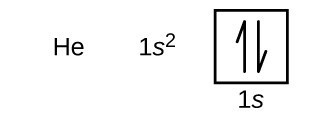The n = 1 shell is completely filled in a helium atom.

The next atom is the alkali metal lithium with an atomic number of 3. The first two electrons in lithium fill the 1s orbital and have the same sets of four quantum numbers as the two electrons in helium. The remaining electron must occupy the orbital of next lowest energy, the 2s orbital (Figure 3 or Figure 4). Thus, the electron configuration and orbital diagram of lithium are:An atom of the alkaline earth metal beryllium, with an atomic number of 4, contains four protons in the nucleus and four electrons surrounding the nucleus. The fourth electron fills the remaining space in the 2s orbital.An atom of boron (atomic number 5) contains five electrons. The n = 1 shell is filled with two electrons and three electrons will occupy the n = 2 shell. Because any s subshell can contain only two electrons, the fifth electron must occupy the next energy level, which will be a 2p orbital. There are three degenerate 2p orbitals (ml = −1, 0, +1) and the electron can occupy any one of these p orbitals. When drawing orbital diagrams, we include empty boxes to depict any empty orbitals in the same subshell that we are filling.Carbon (atomic number 6) has six electrons. Four of them fill the 1s and 2s orbitals. The remaining two electrons occupy the 2p subshell. We now have a choice of filling one of the 2p orbitals and pairing the electrons or of leaving the electrons unpaired in two different, but degenerate, p orbitals. The orbitals are filled as described by Hund’s rule: the lowest-energy configuration for an atom with electrons within a set of degenerate orbitals is that having the maximum number of unpaired electrons. Thus, the two electrons in the carbon 2p orbitals have identical n, l, and ms quantum numbers and differ in their ml quantum number (in accord with the Pauli exclusion principle). The electron configuration and orbital diagram for carbon are: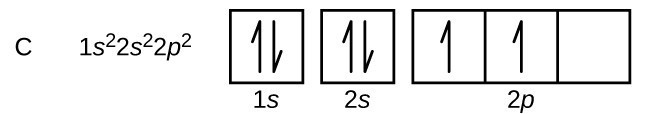Nitrogen (atomic number 7) fills the 1s and 2s subshells and has one electron in each of the three 2p orbitals, in accordance with Hund’s rule. These three electrons have unpaired spins. Oxygen (atomic number 8) has a pair of electrons in any one of the 2p orbitals (the electrons have opposite spins) and a single electron in each of the other two. Fluorine (atomic number 9) has only one 2p orbital containing an unpaired electron. All of the electrons in the noble gas neon (atomic number 10) are paired, and all of the orbitals in the n = 1 and the n = 2 shells are filled. The electron configurations and orbital diagrams of these four elements are: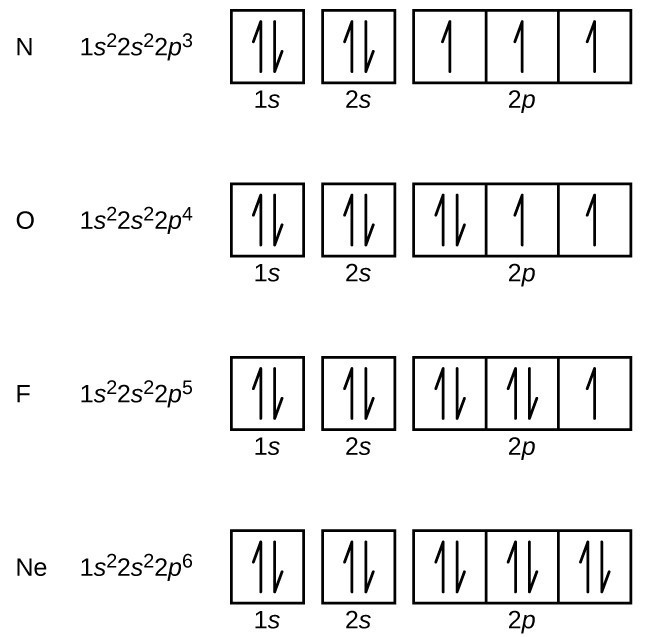The alkali metal sodium (atomic number 11) has one more electron than the neon atom. This electron must go into the lowest-energy subshell available, the 3s orbital, giving a 1s22s22p63s1 configuration. The electrons occupying the outermost shell orbital(s) (highest value of n) are called valence electrons, and those occupying the inner shell orbitals are called core electrons (Figure 5). Since the core electron shells correspond to noble gas electron configurations, we can abbreviate electron configurations by writing the noble gas that matches the core electron configuration, along with the valence electrons in a condensed format. For our sodium example, the symbol [Ne] represents core electrons, (1s22s22p6) and our abbreviated or condensed configuration is [Ne]3s1.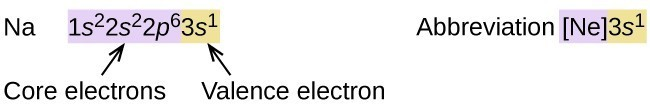Figure 5. A core-abbreviated electron configuration (right) replaces the core electrons with the noble gas symbol whose configuration matches the core electron configuration of the other element.

Similarly, the abbreviated configuration of lithium can be represented as [He]2s1, where [He] represents the configuration of the helium atom, which is identical to that of the filled inner shell of lithium. Writing the configurations in this way emphasizes the similarity of the configurations of lithium and sodium. Both atoms, which are in the alkali metal family, have only one electron in a valence s subshell outside a filled set of inner shells.

$\begin{array}{l}\text{Li:}\left[\text{He}\right]2{s}^{1}\\ \text{Na:}\left[\text{Ne}\right]3{s}^{1}\end{array}$

The alkaline earth metal magnesium (atomic number 12), with its 12 electrons in a [Ne]3s2 configuration, is analogous to its family member beryllium, [He]2s2. Both atoms have a filled s subshell outside their filled inner shells. Aluminum (atomic number 13), with 13 electrons and the electron configuration [Ne]3s23p1, is analogous to its family member boron, [He]2s22p1.

The electron configurations of silicon (14 electrons), phosphorus (15 electrons), sulfur (16 electrons), chlorine (17 electrons), and argon (18 electrons) are analogous in the electron configurations of their outer shells to their corresponding family members carbon, nitrogen, oxygen, fluorine, and neon, respectively, except that the principal quantum number of the outer shell of the heavier elements has increased by one to n = 3. Figure 6 shows the lowest energy, or ground-state, electron configuration for these elements as well as that for atoms of each of the known elements.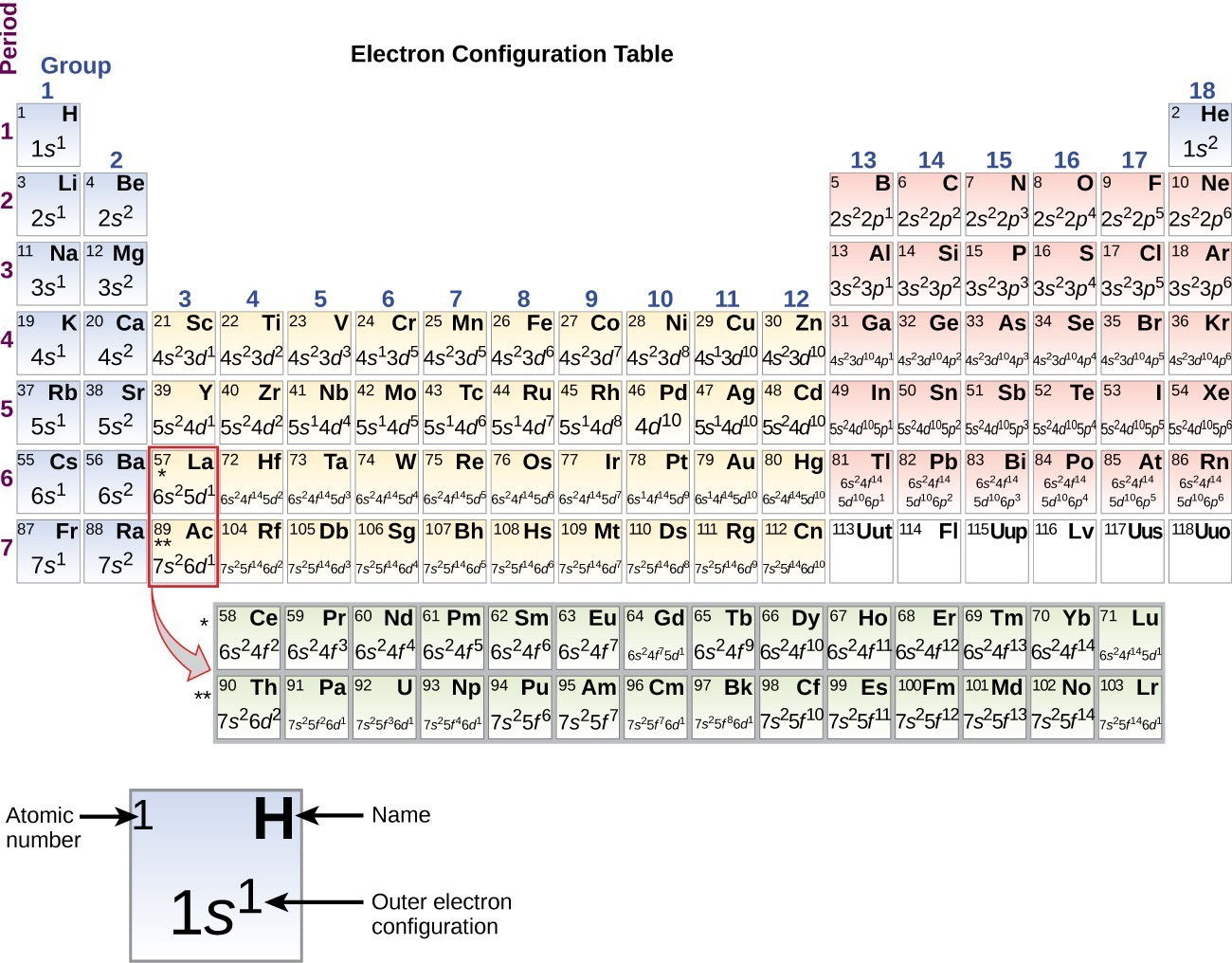Figure 6. This version of the periodic table shows the outer-shell electron configuration of each element. Note that down each group, the configuration is often similar.

When we come to the next element in the periodic table, the alkali metal potassium (atomic number 19), we might expect that we would begin to add electrons to the 3d subshell. However, all available chemical and physical evidence indicates that potassium is like lithium and sodium, and that the next electron is not added to the 3d level but is, instead, added to the 4s level (Figure 6). As discussed previously, the 3d orbital with no radial nodes is higher in energy because it is less penetrating and more shielded from the nucleus than the 4s, which has three radial nodes. Thus, potassium has an electron configuration of [Ar]4s1. Hence, potassium corresponds to Li and Na in its valence shell configuration. The next electron is added to complete the 4s subshell and calcium has an electron configuration of [Ar]4s2. This gives calcium an outer-shell electron configuration corresponding to that of beryllium and magnesium.

Beginning with the transition metal scandium (atomic number 21), additional electrons are added successively to the 3d subshell. This subshell is filled to its capacity with 10 electrons (remember that for l = 2 [d orbitals], there are 2l + 1 = 5 values of ml, meaning that there are five d orbitals that have a combined capacity of 10 electrons). The 4p subshell fills next. Note that for three series of elements, scandium (Sc) through copper (Cu), yttrium (Y) through silver (Ag), and lutetium (Lu) through gold (Au), a total of 10 d electrons are successively added to the (n – 1) shell next to the n shell to bring that (n – 1) shell from 8 to 18 electrons. For two series, lanthanum (La) through lutetium (Lu) and actinium (Ac) through lawrencium (Lr), 14 f electrons (l = 3, 2l + 1 = 7 ml values; thus, seven orbitals with a combined capacity of 14 electrons) are successively added to the (n – 2) shell to bring that shell from 18 electrons to a total of 32 electrons.

### Example 1: Quantum Numbers and Electron Configurations

What is the electron configuration and orbital diagram for a phosphorus atom? What are the four quantum numbers for the last electron added?

Identify the atoms from the electron configurations given:

1. [Ar]4s23d5
2. [Kr]5s24d105p6.

The periodic table can be a powerful tool in predicting the electron configuration of an element. However, we do find exceptions to the order of filling of orbitals that are shown in Figure 3 or Figure 4. For instance, the electron configurations (shown in Figure 6) of the transition metals chromium (Cr; atomic number 24) and copper (Cu; atomic number 29), among others, are not those we would expect. In general, such exceptions involve subshells with very similar energy, and small effects can lead to changes in the order of filling.

In the case of Cr and Cu, we find that half-filled and completely filled subshells apparently represent conditions of preferred stability. This stability is such that an electron shifts from the 4s into the 3d orbital to gain the extra stability of a half-filled 3d subshell (in Cr) or a filled 3d subshell (in Cu). Other exceptions also occur. For example, niobium (Nb, atomic number 41) is predicted to have the electron configuration [Kr]5s24d3. Experimentally, we observe that its ground-state electron configuration is actually [Kr]5s14d4. We can rationalize this observation by saying that the electron–electron repulsions experienced by pairing the electrons in the 5s orbital are larger than the gap in energy between the 5s and 4d orbitals. There is no simple method to predict the exceptions for atoms where the magnitude of the repulsions between electrons is greater than the small differences in energy between subshells.

## Electron Configurations and the Periodic Table

As described earlier, the periodic table arranges atoms based on increasing atomic number so that elements with the same chemical properties recur periodically. When their electron configurations are added to the table (Figure 6), we also see a periodic recurrence of similar electron configurations in the outer shells of these elements. Because they are in the outer shells of an atom, valence electrons play the most important role in chemical reactions. The outer electrons have the highest energy of the electrons in an atom and are most easily lost or shared than the core electrons. Valence electrons are also the determining factor in some physical properties of the elements.

Elements in any one group (or column) have the same number of valence electrons; the alkali metals lithium and sodium each have only one valence electron, the alkaline earth metals beryllium and magnesium each have two, and the halogens fluorine and chlorine each have seven valence electrons. The similarity in chemical properties among elements of the same group occurs because they have the same number of valence electrons. It is the loss, gain, or sharing of valence electrons that defines how elements react.

It is important to remember that the periodic table was developed on the basis of the chemical behavior of the elements, well before any idea of their atomic structure was available. Now we can understand why the periodic table has the arrangement it has—the arrangement puts elements whose atoms have the same number of valence electrons in the same group. This arrangement is emphasized in Figure 6, which shows in periodic-table form the electron configuration of the last subshell to be filled by the Aufbau principle. The colored sections of Figure 6 show the three categories of elements classified by the orbitals being filled: main group, transition, and inner transition elements. These classifications determine which orbitals are counted in the valence shell, or highest energy level orbitals of an atom.

1. Main group elements (sometimes called representative elements) are those in which the last electron added enters an s or a p orbital in the outermost shell, shown in blue and red in Figure 6. This category includes all the nonmetallic elements, as well as many metals and the intermediate semimetallic elements. The valence electrons for main group elements are those with the highest n level. For example, gallium (Ga, atomic number 31) has the electron configuration [Ar]4s23d104p1, which contains three valence electrons (underlined). The completely filled d orbitals count as core, not valence, electrons.
2. Transition elements or transition metals. These are metallic elements in which the last electron added enters a d orbital. The valence electrons (those added after the last noble gas configuration) in these elements include the ns and (n – 1) d electrons. The official IUPAC definition of transition elements specifies those with partially filled d orbitals. Thus, the elements with completely filled orbitals (Zn, Cd, Hg, as well as Cu, Ag, and Au in Figure 6) are not technically transition elements. However, the term is frequently used to refer to the entire d block (colored yellow in Figure 6), and we will adopt this usage in this textbook.
3. Inner transition elements are metallic elements in which the last electron added occupies an f orbital. They are shown in green in Figure 6. The valence shells of the inner transition elements consist of the (n – 2)f, the (n – 1)d, and the ns subshells. There are two inner transition series:
1. The lanthanide series: lanthanide (La) through lutetium (Lu)
2. The actinide series: actinide (Ac) through lawrencium (Lr)

Lanthanum and actinium, because of their similarities to the other members of the series, are included and used to name the series, even though they are transition metals with no f electrons.

## Electron Configurations of Ions

We have seen that ions are formed when atoms gain or lose electrons. A cation (positively charged ion) forms when one or more electrons are removed from a parent atom. For main group elements, the electrons that were added last are the first electrons removed. For transition metals and inner transition metals, however, electrons in the s orbital are easier to remove than the d  or f  electrons, and so the  highest ns electrons are lost, and then the (n – 1)d or  (n – 2)f electrons are removed. An anion (negatively charged ion) forms when one or more electrons are added to a parent atom. The added electrons fill in the order predicted by the Aufbau principle.

### Example 2: Predicting Electron Configurations of Ions

What is the electron configuration and orbital diagram of:

1. Na+
2. P3–
3. Al2+
4. Fe2+
5. Sm3+

Which ion with a +2 charge has the electron configuration 1s22s22p63s23p63d104s24p64d5? Which ion with a +3 charge has this configuration?

### Key Concepts and Summary

The relative energy of the subshells determine the order in which atomic orbitals are filled (1s, 2s, 2p, 3s, 3p, 4s, 3d, 4p, and so on). Electron configurations and orbital diagrams can be determined by applying the Pauli exclusion principle (no two electrons can have the same set of four quantum numbers) and Hund’s rule (whenever possible, electrons retain unpaired spins in degenerate orbitals).

Electrons in the outermost orbitals, called valence electrons, are responsible for most of the chemical behavior of elements. In the periodic table, elements with analogous valence electron configurations usually occur within the same group. There are some exceptions to the predicted filling order, particularly when half-filled or completely filled orbitals can be formed. The periodic table can be divided into three categories based on the orbital in which the last electron to be added is placed: main group elements (s and p orbitals), transition elements (d orbitals), and inner transition elements (f orbitals).

### Exercises

1. Read the labels of several commercial products and identify monatomic ions of at least four transition elements contained in the products. Write the complete electron configurations of these cations.
2. Read the labels of several commercial products and identify monatomic ions of at least six main group elements contained in the products. Write the complete electron configurations of these cations and anions.
3. Using complete subshell notation (not abbreviations, 1s22s22p6, and so forth), predict the electron configuration of each of the following atoms:
1. C
2. P
3. V
4. Sb
5. Sm
4. Using complete subshell notation (1s22s22p6, and so forth), predict the electron configuration of each of the following atoms:
1. N
2. Si
3. Fe
4. Te
5. Tb
5. Is 1s22s22p6 the symbol for a macroscopic property or a microscopic property of an element? Explain your answer.
6. What additional information do we need to answer the question “Which ion has the electron configuration 1s22s22p63s23p6”?
7. Draw the orbital diagram for the valence shell of each of the following atoms:
1.  C
2. P
3. V
4. Sb
5. Ru
8. Use an orbital diagram to describe the electron configuration of the valence shell of each of the following atoms:
1. N
2. Si
3. Fe
4. Te
5. Mo
9. Using complete subshell notation (1s22s22p6, and so forth), predict the electron configurations of the following ions.
1. N3–
2. Ca2+
3. S–
4. Cs2+
5. Cr2+
6. Gd3+
10. Which atom has the electron configuration 1s22s22p63s23p64s23d104p65s24d2?
11. Which atom has the electron configuration 1s22s22p63s23p63d74s2?
12. Which ion with a +1 charge has the electron configuration 1s22s22p63s23p63d104s24p6? Which ion with a –2 charge has this configuration?
13. Which of the following atoms contains only three valence electrons: Li, B, N, F, Ne?
14. Which of the following has two unpaired electrons?
1. Mg
2. Si
3. S
4. Both Mg and S
5. Both Si and S.
15. Which atom would be expected to have a half-filled 6p subshell?
16. Which atom would be expected to have a half-filled 4s subshell?
17. In one area of Australia, the cattle did not thrive despite the presence of suitable forage. An investigation showed the cause to be the absence of sufficient cobalt in the soil. Cobalt forms cations in two oxidation states, Co2+ and Co3+. Write the electron structure of the two cations.
18. Thallium was used as a poison in the Agatha Christie mystery story “The Pale Horse.” Thallium has two possible cationic forms, +1 and +3. The +1 compounds are the more stable. Write the electron structure of the +1 cation of thallium.
19. Write the electron configurations for the following atoms or ions:
1. B3+
2. O–
3. Cl3+
4. Ca2+
5. Ti
20. Cobalt–60 and iodine–131 are radioactive isotopes commonly used in nuclear medicine. How many protons, neutrons, and electrons are in atoms of these isotopes? Write the complete electron configuration for each isotope.
21. Write a set of quantum numbers for each of the electrons with an n of 3 in a Sc atom.

## Glossary

Aufbau principle: procedure in which the electron configuration of the elements is determined by “building” them in order of atomic numbers, adding one proton to the nucleus and one electron to the proper subshell at a time

core electron: electron in an atom that occupies the orbitals of the inner shells

electron configuration: electronic structure of an atom in its ground state given as a listing of the orbitals occupied by the electrons

Hund’s rule: every orbital in a subshell is singly occupied with one electron before any one orbital is doubly occupied, and all electrons in singly occupied orbitals have the same spin

orbital diagram: pictorial representation of the electron configuration showing each orbital as a box and each electron as an arrow

valence electrons: electrons in the outermost or valence shell (highest value of n) of a ground-state atom; determine how an element reacts

valence shell: outermost shell of electrons in a ground-state atom; for main group elements, the orbitals with the highest n level (s and p subshells) are in the valence shell, while for transition metals, the highest energy s and d subshells make up the valence shell and for inner transition elements, the highest s, d, and f subshells are included